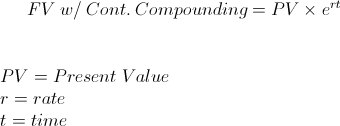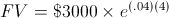# FV - Continuous Compounding

Future Value Continuous Compounding Calculator (Click Here or Scroll Down)The future value with continuous compounding formula is used in calculating the later value of a current sum of money. Use of the future value with continuous compounding formula requires understanding of 3 general financial concepts, which are time value of money, future value as it applies to the time value of money, and continuous compounding.

## Time Value of Money, Future Value, and Continuous Compounding

Time Value of Money - The future value with continuous compounding formula relies on the underlying concept of time value of money. Time value of money is the notion that a current sum of money(or unit of account) is worth more today than the same amount at a future date.

Future Value - Future value expands upon the idea of time value of money in that it quantifies the amount required at a later date. For example, suppose that an individual has a choice between receiving \$1000 today or \$1050 one year from today. Is the additional \$50 worth waiting one year for? Can the individual invest elsewhere and make a higher return? Could the individual use the \$1000 now for a higher "utility of enjoyment" than the \$50 warrants? The future value with continuous compounding formula calculates the later value when there is continuous compounding.

Continuous Compounding - Continuous compounding is compounding that is in constant motion as opposed to incremental steps. Continuous compounding is considered to have an infinite amount of compounding periods for a certain period of time because there is no incremental steps as found in monthly or annual compounding.

Particularly the last 2 of these concepts lends to the actual formula for future value with continuous compounding.

## Example of FV with Continuous Compounding Formula

An example of the future value with continuous compounding formula is an individual would like to calculate the balance of her account after 4 years which earns 4% per year, continuously compounded, if she currently has a balance of \$3000.

The variables for this example would be 4 for time, t, .04 for the rate, r, and the present value would be \$3000. The equation for this example would bewhich return a result of \$3520.53.

New to Finance?